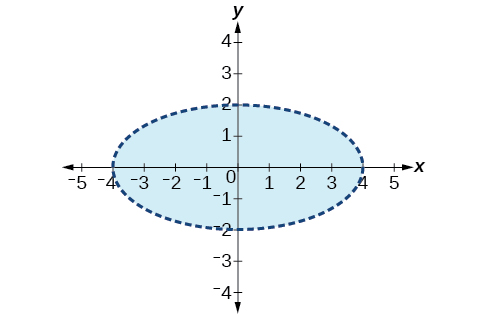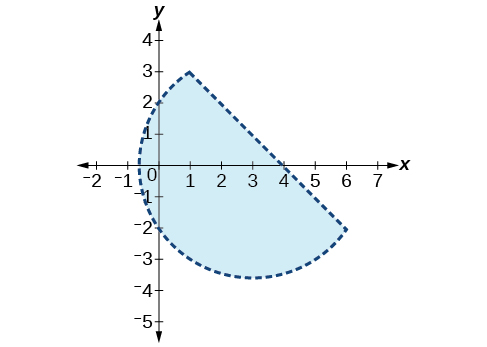# 11.8 Solving systems with cramer's rule  (Page 8/11)

 Page 8 / 11

$\left(10,-10,10\right)$

$\begin{array}{r}\hfill 5x+3y-z=5\text{\hspace{0.17em}}\text{\hspace{0.17em}}\text{\hspace{0.17em}}\\ \hfill 3x-2y+4z=13\\ \hfill 4x+3y+5z=22\end{array}$

$\begin{array}{r}x+y+z=1\\ 2x+2y+2z=1\\ 3x+3y=2\end{array}$

No solutions exist.

$\begin{array}{l}\text{\hspace{0.17em}}\text{\hspace{0.17em}}3x+2y-z=-10\hfill \\ \text{\hspace{0.17em}}\text{\hspace{0.17em}}\text{\hspace{0.17em}}\text{\hspace{0.17em}}x-y+2z=7\hfill \\ -x+3y+z=-2\hfill \end{array}$

$\left(-1,-2,3\right)$

$\begin{array}{r}\hfill 3x+4z=-11\\ \hfill x-2y=5\text{\hspace{0.17em}}\text{\hspace{0.17em}}\text{\hspace{0.17em}}\text{\hspace{0.17em}}\text{\hspace{0.17em}}\text{\hspace{0.17em}}\text{\hspace{0.17em}}\\ \hfill 4y-z=-10\end{array}$

$\begin{array}{r}2x-3y+z=0\\ 2x+4y-3z=0\\ 6x-2y-z=0\end{array}$

$\left(x,\frac{8x}{5},\frac{14x}{5}\right)$

$\begin{array}{r}6x-4y-2z=2\\ 3x+2y-5z=4\\ 6y-7z=5\end{array}$

For the following exercises, write a system of equations to solve each problem. Solve the system of equations.

Three odd numbers sum up to 61. The smaller is one-third the larger and the middle number is 16 less than the larger. What are the three numbers?

11, 17, 33

A local theatre sells out for their show. They sell all 500 tickets for a total purse of $8,070.00. The tickets were priced at$15 for students, $12 for children, and$18 for adults. If the band sold three times as many adult tickets as children’s tickets, how many of each type was sold?

## Systems of Nonlinear Equations and Inequalities: Two Variables

For the following exercises, solve the system of nonlinear equations.

$\begin{array}{l}\begin{array}{l}\\ y={x}^{2}-7\end{array}\hfill \\ y=5x-13\hfill \end{array}$

$\left(2,-3\right),\left(3,2\right)$

$\begin{array}{l}\begin{array}{l}\\ y={x}^{2}-4\end{array}\hfill \\ y=5x+10\hfill \end{array}$

$\begin{array}{l}{x}^{2}+{y}^{2}=16\hfill \\ \text{\hspace{0.17em}}\text{\hspace{0.17em}}\text{\hspace{0.17em}}\text{\hspace{0.17em}}\text{\hspace{0.17em}}\text{\hspace{0.17em}}\text{\hspace{0.17em}}\text{\hspace{0.17em}}\text{\hspace{0.17em}}\text{\hspace{0.17em}}\text{\hspace{0.17em}}\text{\hspace{0.17em}}\text{\hspace{0.17em}}\text{\hspace{0.17em}}\text{\hspace{0.17em}}\text{\hspace{0.17em}}y=x-8\hfill \end{array}$

No solution

$\begin{array}{l}{x}^{2}+{y}^{2}=25\hfill \\ \text{\hspace{0.17em}}\text{\hspace{0.17em}}\text{\hspace{0.17em}}\text{\hspace{0.17em}}\text{\hspace{0.17em}}\text{\hspace{0.17em}}\text{\hspace{0.17em}}\text{\hspace{0.17em}}\text{\hspace{0.17em}}\text{\hspace{0.17em}}\text{\hspace{0.17em}}\text{\hspace{0.17em}}\text{\hspace{0.17em}}\text{\hspace{0.17em}}\text{\hspace{0.17em}}\text{\hspace{0.17em}}y={x}^{2}+5\hfill \end{array}$

$\begin{array}{r}{x}^{2}+{y}^{2}=4\\ y-{x}^{2}=3\end{array}$

No solution

For the following exercises, graph the inequality.

$y>{x}^{2}-1$

$\frac{1}{4}{x}^{2}+{y}^{2}<4$For the following exercises, graph the system of inequalities.

$\begin{array}{l}{x}^{2}+{y}^{2}+2x<3\hfill \\ \text{\hspace{0.17em}}\text{\hspace{0.17em}}\text{\hspace{0.17em}}\text{\hspace{0.17em}}\text{\hspace{0.17em}}\text{\hspace{0.17em}}\text{\hspace{0.17em}}\text{\hspace{0.17em}}\text{\hspace{0.17em}}\text{\hspace{0.17em}}\text{\hspace{0.17em}}\text{\hspace{0.17em}}\text{\hspace{0.17em}}\text{\hspace{0.17em}}\text{\hspace{0.17em}}\text{\hspace{0.17em}}\text{\hspace{0.17em}}\text{\hspace{0.17em}}\text{\hspace{0.17em}}\text{\hspace{0.17em}}\text{\hspace{0.17em}}\text{\hspace{0.17em}}\text{\hspace{0.17em}}\text{\hspace{0.17em}}\text{\hspace{0.17em}}\text{\hspace{0.17em}}\text{\hspace{0.17em}}\text{\hspace{0.17em}}\text{\hspace{0.17em}}y>-{x}^{2}-3\hfill \end{array}$

$\begin{array}{l}{x}^{2}-2x+{y}^{2}-4x<4\hfill \\ \text{\hspace{0.17em}}\text{\hspace{0.17em}}\text{\hspace{0.17em}}\text{\hspace{0.17em}}\text{\hspace{0.17em}}\text{\hspace{0.17em}}\text{\hspace{0.17em}}\text{\hspace{0.17em}}\text{\hspace{0.17em}}\text{\hspace{0.17em}}\text{\hspace{0.17em}}\text{\hspace{0.17em}}\text{\hspace{0.17em}}\text{\hspace{0.17em}}\text{\hspace{0.17em}}\text{\hspace{0.17em}}\text{\hspace{0.17em}}\text{\hspace{0.17em}}\text{\hspace{0.17em}}\text{\hspace{0.17em}}\text{\hspace{0.17em}}\text{\hspace{0.17em}}\text{\hspace{0.17em}}\text{\hspace{0.17em}}\text{\hspace{0.17em}}\text{\hspace{0.17em}}\text{\hspace{0.17em}}\text{\hspace{0.17em}}\text{\hspace{0.17em}}\text{\hspace{0.17em}}\text{\hspace{0.17em}}\text{\hspace{0.17em}}\text{\hspace{0.17em}}\text{\hspace{0.17em}}\text{\hspace{0.17em}}\text{\hspace{0.17em}}\text{\hspace{0.17em}}\text{\hspace{0.17em}}\text{\hspace{0.17em}}\text{\hspace{0.17em}}\text{\hspace{0.17em}}\text{\hspace{0.17em}}y<-x+4\hfill \end{array}$$\begin{array}{l}{x}^{2}+{y}^{2}<1\hfill \\ \text{\hspace{0.17em}}\text{\hspace{0.17em}}\text{\hspace{0.17em}}\text{\hspace{0.17em}}\text{\hspace{0.17em}}\text{\hspace{0.17em}}\text{\hspace{0.17em}}\text{\hspace{0.17em}}\text{\hspace{0.17em}}\text{\hspace{0.17em}}\text{\hspace{0.17em}}\text{\hspace{0.17em}}\text{\hspace{0.17em}}{y}^{2}

## Partial Fractions

For the following exercises, decompose into partial fractions.

$\frac{-2x+6}{{x}^{2}+3x+2}$

$\frac{2}{x+2},\frac{-4}{x+1}$

$\frac{10x+2}{4{x}^{2}+4x+1}$

$\frac{7x+20}{{x}^{2}+10x+25}$

$\frac{7}{x+5},\frac{-15}{{\left(x+5\right)}^{2}}$

$\frac{x-18}{{x}^{2}-12x+36}$

$\frac{-{x}^{2}+36x+70}{{x}^{3}-125}$

$\frac{3}{x-5},\frac{-4x+1}{{x}^{2}+5x+25}$

$\frac{-5{x}^{2}+6x-2}{{x}^{3}+27}$

$\frac{{x}^{3}-4{x}^{2}+3x+11}{{\left({x}^{2}-2\right)}^{2}}$

$\frac{x-4}{\left({x}^{2}-2\right)},\frac{5x+3}{{\left({x}^{2}-2\right)}^{2}}$

$\frac{4{x}^{4}-2{x}^{3}+22{x}^{2}-6x+48}{x{\left({x}^{2}+4\right)}^{2}}$

## Matrices and Matrix Operations

For the following exercises, perform the requested operations on the given matrices.

$A=\left[\begin{array}{rr}\hfill 4& \hfill -2\\ \hfill 1& \hfill 3\end{array}\right],B=\left[\begin{array}{rrr}\hfill 6& \hfill 7& \hfill -3\\ \hfill 11& \hfill -2& \hfill 4\end{array}\right],C=\left[\begin{array}{r}\hfill \begin{array}{cc}6& 7\\ 11& -2\end{array}\\ \hfill \begin{array}{cc}14& 0\end{array}\end{array}\right],D=\left[\begin{array}{rrr}\hfill 1& \hfill -4& \hfill 9\\ \hfill 10& \hfill 5& \hfill -7\\ \hfill 2& \hfill 8& \hfill 5\end{array}\right],E=\left[\begin{array}{rrr}\hfill 7& \hfill -14& \hfill 3\\ \hfill 2& \hfill -1& \hfill 3\\ \hfill 0& \hfill 1& \hfill 9\end{array}\right]$

$-4A$

$\left[\begin{array}{cc}-16& 8\\ -4& -12\end{array}\right]$

$10D-6E$

$B+C$

undefined; dimensions do not match

$AB$

$BA$

undefined; inner dimensions do not match

$BC$

$CB$

$\left[\begin{array}{ccc}113& 28& 10\\ 44& 81& -41\\ 84& 98& -42\end{array}\right]$

$DE$

$ED$

$\left[\begin{array}{ccc}-127& -74& 176\\ -2& 11& 40\\ 28& 77& 38\end{array}\right]$

$EC$

$CE$

undefined; inner dimensions do not match

${A}^{3}$

## Solving Systems with Gaussian Elimination

For the following exercises, write the system of linear equations from the augmented matrix. Indicate whether there will be a unique solution.

$\begin{array}{l}x-3z=7\\ y+2z=-5\text{\hspace{0.17em}}\end{array}$ with infinite solutions

For the following exercises, write the augmented matrix from the system of linear equations.

$\begin{array}{l}\\ \begin{array}{r}\hfill -2x+2y+z=7\\ \hfill 2x-8y+5z=0\\ \hfill 19x-10y+22z=3\end{array}\end{array}$

$\begin{array}{l}\text{\hspace{0.17em}}\text{\hspace{0.17em}}\text{\hspace{0.17em}}\text{\hspace{0.17em}}\text{\hspace{0.17em}}4x+2y-3z=14\hfill \\ -12x+3y+z=100\hfill \\ \text{\hspace{0.17em}}\text{\hspace{0.17em}}\text{\hspace{0.17em}}\text{\hspace{0.17em}}\text{\hspace{0.17em}}9x-6y+2z=31\hfill \end{array}$

$\begin{array}{r}\hfill x+3z=12\text{\hspace{0.17em}}\\ \hfill -x+4y=0\text{\hspace{0.17em}}\text{\hspace{0.17em}}\text{\hspace{0.17em}}\text{\hspace{0.17em}}\\ \hfill y+2z=-7\end{array}$

For the following exercises, solve the system of linear equations using Gaussian elimination.

$\begin{array}{r}3x-4y=-7\\ -6x+8y=14\end{array}$

$\begin{array}{r}3x-4y=1\\ -6x+8y=6\end{array}$

No solutions exist.

$\begin{array}{l}\begin{array}{l}\\ -1.1x-2.3y=6.2\end{array}\hfill \\ -5.2x-4.1y=4.3\hfill \end{array}$

$\begin{array}{r}\hfill 2x+3y+2z=1\text{\hspace{0.17em}}\text{\hspace{0.17em}}\text{\hspace{0.17em}}\text{\hspace{0.17em}}\text{\hspace{0.17em}}\\ \hfill -4x-6y-4z=-2\\ \hfill 10x+15y+10z=0\text{\hspace{0.17em}}\text{\hspace{0.17em}}\text{\hspace{0.17em}}\text{\hspace{0.17em}}\text{\hspace{0.17em}}\end{array}$

No solutions exist.

$\begin{array}{r}\hfill -x+2y-4z=8\text{\hspace{0.17em}}\text{\hspace{0.17em}}\text{\hspace{0.17em}}\text{\hspace{0.17em}}\\ \hfill 3y+8z=-4\\ \hfill -7x+y+2z=1\text{\hspace{0.17em}}\text{\hspace{0.17em}}\text{\hspace{0.17em}}\text{\hspace{0.17em}}\end{array}$

## Solving Systems with Inverses

For the following exercises, find the inverse of the matrix.

$\left[\begin{array}{rr}\hfill -0.2& \hfill 1.4\\ \hfill 1.2& \hfill -0.4\end{array}\right]$

$\frac{1}{8}\left[\begin{array}{cc}2& 7\\ 6& 1\end{array}\right]$

$\left[\begin{array}{rr}\hfill \frac{1}{2}& \hfill -\frac{1}{2}\\ \hfill -\frac{1}{4}& \hfill \frac{3}{4}\end{array}\right]$

$\left[\begin{array}{ccc}12& 9& -6\\ -1& 3& 2\\ -4& -3& 2\end{array}\right]$

No inverse exists.

$\left[\begin{array}{ccc}2& 1& 3\\ 1& 2& 3\\ 3& 2& 1\end{array}\right]$

#### Questions & Answers

write down the polynomial function with root 1/3,2,-3 with solution
if A and B are subspaces of V prove that (A+B)/B=A/(A-B)
write down the value of each of the following in surd form a)cos(-65°) b)sin(-180°)c)tan(225°)d)tan(135°)
Prove that (sinA/1-cosA - 1-cosA/sinA) (cosA/1-sinA - 1-sinA/cosA) = 4
what is the answer to dividing negative index
In a triangle ABC prove that. (b+c)cosA+(c+a)cosB+(a+b)cisC=a+b+c.
give me the waec 2019 questions
the polar co-ordinate of the point (-1, -1)
prove the identites sin x ( 1+ tan x )+ cos x ( 1+ cot x )= sec x + cosec x
tanh`(x-iy) =A+iB, find A and B
B=Ai-itan(hx-hiy)
Rukmini
what is the addition of 101011 with 101010
If those numbers are binary, it's 1010101. If they are base 10, it's 202021.
Jack
extra power 4 minus 5 x cube + 7 x square minus 5 x + 1 equal to zero
the gradient function of a curve is 2x+4 and the curve passes through point (1,4) find the equation of the curve
1+cos²A/cos²A=2cosec²A-1
test for convergence the series 1+x/2+2!/9x3ByByBy Gerr ZenBy RhodesByBy